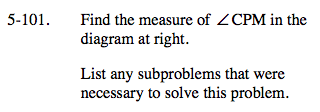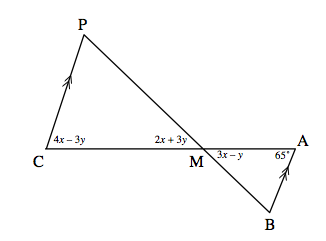### Home > A2C > Chapter 5 > Lesson 5.2.4 > Problem5-101

5-101.$\text{Notice that }\overline{AB} \text{ and }\overline{CP} \text{ are parallel.}$

Remember geometric angle relationships.
If the lines are parallel, what must be true about the alternate interior angles?
What is always true about vertical angles?

65 = 4x − 3y
3xy = 2x + 3y

How can you find the measure of the third angle?Write and solve a system of equations based on the properties.

Once you find x and y, find the measures of the angles in ΔCPM.

CPM = 60°